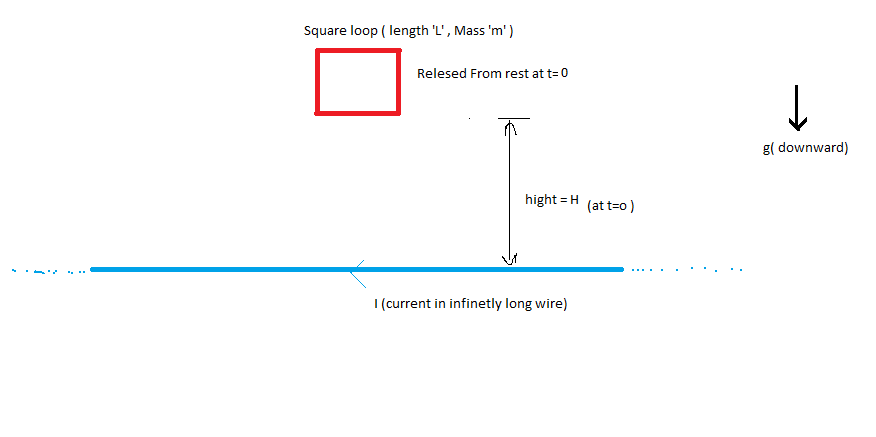# Magnetism + Calculus + Mechanics = MegatulicsLet an Super Conducting Square Loop ( having negligible resistance ) and has Self inductance "L" and has mass of "m" and side length $l$ is released from rest in the gravity from the height "H" at time t=0.There is an infinitely Long current carrying wire which is in Same Plane of that of Square Loop and current ${ I }_{ 0 }$ flowing in it.

Then Find The Velocity of the square loop when it is at height of $\frac { H }{ 4 }$ from the wire.

Details and assumptions

$\bullet \quad m=1\quad kg\\ \bullet \quad L=10\quad henry\\ \bullet \quad l=1m\\ \bullet \quad { I }_{ 0 }={ 10 }^{ 7 }\quad Amp.\\ \bullet \quad H=2\quad m\\ \bullet \quad g=9.8\quad m/{ s }^{ 2 }$.

×